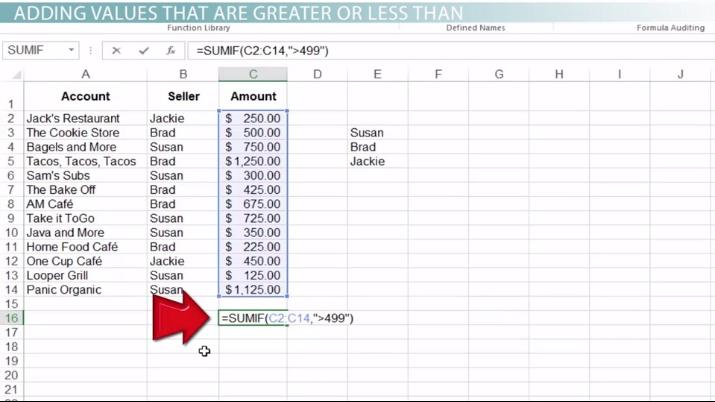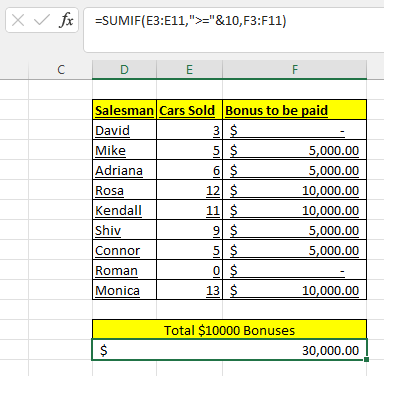# How to use the SUMIF Function in Excel

Marco Antonio Hernandez Cardona, Karen Sorensen
• Author
Marco Antonio Hernandez Cardona

Marco has taught elementary school, high school and college (math, physics, chemistry, ecology, calculus, and other science subjects) for over 3 years. He is a civil engineer with a MEng degree on Environmental Engineering in the Yucatan Autonomous University (UADY) in Mérida, México

• Instructor
Karen Sorensen

Karen has a Bachelors in Communications. She has 25 years of experience in Information Systems, Adult Learning and Virtual Training.

What is a SUMIF Function and how it is used in Excel? Learn the formula and syntax of the SUMIF function. Understand how to use the SUMIF function in Excel. Updated: 11/23/2021

Show

## What is a SUMIF Function in Excel?

The SUMIF Function in Excel is used to sum the the range values in a range that meet a specified criteria. For example, having a spreadsheet with information about the money spent in certain month, the user can apply this function to sum only the values that correspond to a specific month.An error occurred trying to load this video.

Try refreshing the page, or contact customer support.

Coming up next: How to Use the AVERAGEIF Function in Excel

### You're on a roll. Keep up the good work!

Replay
Your next lesson will play in 10 seconds
• 0:02 SUMIF
• 0:30 SUMIF in a Formula
• 1:34 Values Greater or Less Than
• 2:45 Where the Criteria Is Text
• 4:31 More Example Formulas
• 5:50 Lesson Summary
Save Save

Want to watch this again later?

Timeline
Autoplay
Autoplay
Speed Speed

## Syntax of SUMIF Function

The SUMIF Function follows this structure:

{eq}\text {=SUMIF(Range, Criteria, Sum_Range)} {/eq}

Basically, what the formula shows is this :

=SUMIF (In which range of cells is Excel going to find the data that meets the criteria?, What criteria must the data comply with?,What values is Excel going to SUMIF the condition is true?)

If the first cell in the range meets the proposed condition, Excel will SUM the corresponding value. If the first cell in the range does not meet the proposed condition, Excel doesn't SUM anything. The function then continues to the next cell, and so on and so forth until it reaches the last cell of the range. This means that the result of the formula is the SUM of all the corresponding values that met the criteria.

### Arguments

This function includes three arguments: the range, the criteria the cells need to fulfill, and the range of values to SUM if the criteria is fulfilled.

#### Range

The range is the list of cells that the function is going to evaluate and compare with the given criteria. The range can include rows, columns, or a matrix of rows and columns.

#### Criteria

The criteria is the condition that the cell in the selected range must follow to be considered TRUE, and to add +1 to the counter. This condition can be expressed through logical operators or wildcards.

 Logical Operators Condition TRUE Condition Examples = Equal to 3=3 (three is equal to three) < Less than 3<4 (three is less than 4) > Greater than 3>2 (three is greater than 2) <> Not equal to 3<>2 (three is not equal to 2) <= Less than or equal to 3<=3 (three is less than or equal to three) >= Greather than or equal to 3>=3 (three is greather than or equal to three)

Another feature that Excel supports is the use of wildcards to find a specific condition within the cells of the spreadsheet.

 Wildcard Condition Examples * Counts the number of cells that contain one or various alphanumeric characters "*Green*" (Is TRUE for cells that contain the word "Green"), "1*4" (Is True for cells that contain the 1 and 4 numbers), "*s"(Is true for cells that cointain words ending with S), "s*"(Is true for cells that Start with S) ? Counts the number of cells that contain a unique alphanumeric character. It doesn't matter where it is located in the cell "Green?"(Is true for cells that contain the unique word Green), "S?T" (Is true for cells that Start with S and end with T like "SAT" or "SIT", but false for "SUM") ~ Is used when the characters in cells you want to compare contain the characters "*" or "?" "*~?" (Is true when the cells containt text that ends in "?"), "~*" (Is true if the cell cointains the exact character "*")

#### Sum Range

These are the cells that are going to be added. If it is specified in the arguments of the formula, the function will add the values in the range that meet the criteria. If the Sum range is not specified in the formula, then Excel will add all the values in the range. The Sum Range must be the same size of the Range. If the Range covers 10 cells, the sum range needs to cover also a range of 10 cells.

## Uses of the SUMIF Function in Excel

Sometimes the user has values in a range of cells, but only wants to sum some of them, not all. This values to sum need to coincide with an specific condition. This condition can be a text condition, numeric condition or a date. The general process that goes into applying the function formula is the same for all kinds of data:

1. For every Excel function, the user must write the "=" symbol to start introducing the formula
2. Then the user must write the name of the function -- in this case: =SUMIF
3. The function's arguments are written between parenthesis: =SUMIF(Range, Criteria,Sum_range)
4. To select the range, the user can either type the start and end cells directly into the formula bar, or they can click the first cell and drag their cursor to the end of the range to select the whole thing.
5. The criteria is specific to the situation we have and the information we want to extract, but it must always be written between quotation marks, like so: " ". Further sections of the lesson will provide more information about how to format the criteria.
6. The sum range must be selected the same way as the range, and it has to be the same size of that range.

The SUMIF Function is different to the SUMIFS Function because the first one allows to compare the data in the range with only one criteria, and the second one allows to compare with more than one criteria.

### Using the SUMIF Function with Numeric Criteria

In many cases, the user will need to sum values in a range that meet with a numeric condition. Consider the following situation: One store is awarding bonuses of $5,000 to salespeople who sell 5 or more cars and$ 10,000 to those who sell 10 or more. The manager has an Excel spreadsheet with the information about how many cars the salespeople sold, and how much in bonuses each one will receive. He wants to know how much money in $10,000 bonuses he needs to ask for, because these are the first bonuses to be paid. He applies the SUMIF Function to find out that data.The Range of cells where the information about how many cars the salespeople sold is between the cells E3:E11, the logical operator that expresses what the user needs to find is ">=". The sum range is between the cells F3:F11 and it is the same size as the range. When using COUNTIF for numeric data, the syntax changes -- first, the logical operator must be placed between quotation marks, and second, the user must write the ampersand symbol (&) to make a reference to a cell that cointains a numeric value. It is important to write the quotation marks on both sides of the logical operator, so that Excel doesn't take the ampersand symbol as part of the actual criteria. Taking into account all of this information, the resultant formula is: =SUMIF(E3:E11,">="&10, F3:F11) Here is what this formula expresses: Here is what this formula expresses: When the values in the range E3:E11 contain are greater or equal to 10, add the corresponding value in the range F3:F121and give me the total sum". So the result is$30,000.

To unlock this lesson you must be a Study.com Member.

#### How does the Sumif function work?

The user provides a range of values to compare with a specified criteria. He also provides a sum range (Values that are going to be added if the condition is true. In the first cell of the range if the condition is true, the corresponding value is added, if it is FALSE it adds nothing. And this continues through all the cells in the range.

#### What is the function of Sumifs in Excel?

The SUMIFS function in Excel is different to the SUMIF function because it compares the values in the range with two or more criterias. The SUMIF function only allows one criteria to compare with.

### Register to view this lesson

Are you a student or a teacher?